## A 5.00-kg mass on the end of a light string rotates in a circular motion on a horizontal frictionless desk. The radius of the circle is 0.80

Question

A 5.00-kg mass on the end of a light string rotates in a circular motion on a horizontal frictionless desk. The radius of the circle is 0.800 m. The string has a breaking strength of 50.0 N. What is the fastest rotational speed of the mass that will not break the string?

in progress 0
2 months 2021-08-15T05:30:48+00:00 1 Answers 0 views 0

The fastest rotational speed of the mass is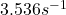.

Explanation:

Here the string’s breaking strength of 50.0 N means that the centripetal force exerted on the 5.00kg mass cannot exceed 50.0N—if it does, the string would break.

Therefore, we demand that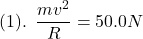where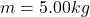,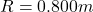is the radius of the circle (also the length of the string), and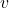is the tangential velocity of the mass.

Now, the tangential velocity can be written in terms of the rotational speed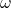as follows: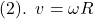,

and putting that into equation (1) we get: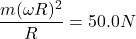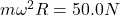,

and we solve for the rotational speedto get: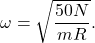Finally, we out in the numeral values and get;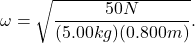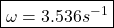which is the fastest rotational speed of the mass.